# Move over VLOOKUP, here comes XLOOKUP

in excel •  10 months ago  (edited)

XLOOKUP Excel 365 (insider edition)

VLOOKUP is one of those functions’ users tend to use over and over.  At the start it is hard to get your head around it but once you do, it provides great value. However, it also has its limitations. For example, on its own it cannot look up to the left.  You cannot do a horizontal lookup. You cannot add new columns to the dataset for fear of breaking the formula.

XLOOKUP aims to solve the limitations posed by VLOOKUP, it will be easier to read and write and it will calculate faster. Now this function is only available on the insiders track but it will be rolled out to 365 soon.

## Move over VLOOKUP

We have covered VLOOKUP here.  We also covered solutions to the limitations here and here.  And we also looked at alternatives such as CHOOSE.  By now you should be familiar with VLOOKUP.  But just to refresh your memory the syntax for VLOOKUP is:

=VLOOKUP(lookup_value, table_array, col_index, [range_lookup])

Where the

lookup_value is the value you wish to look up.

Table array is the table that contains the lookup value .  The lookup value column must be the column to the far most left of the table

Col_index is the column number in the table from which you wish to return a value from.

Range_lookup is the match type.  You can select between an approximate match, which is the default setting, or an exact match.

VLOOKUP works by looking down the first column of the table array until it finds the row with the lookup value.  It then counts the specified number of columns away and returns the value from that cell.

Consider the following table of data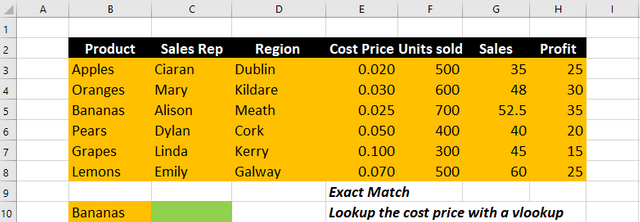We wish to carry out a VLOOKUP to return the cost price of Bananas.  This is the formula we would use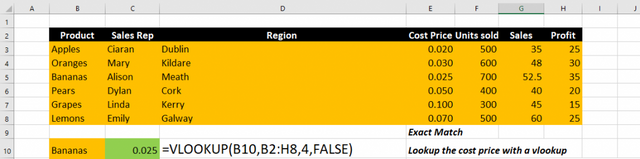Now, you can forget all that!!!!!!!!!!!!!!!!!!

## Here comes XLOOKUP

The syntax for XLOOKUP is

=XLOOKUP(lookup_value, lookup_array, return_array, [match_mode] ,[search_mode])

Ok so XLOOKUP looks a little scarier than VLOOKUP, but once you understand it, you will see how easy it is to use.  The last two parameter in the function are optional( [match_mode], [search_mode]).  That means they can be left out.  So to carry out a look up with an exact match you only need the first 3 parameters.  Therefore, you can use

=XLOOKUP(lookup_value, lookup_array, return_array)

Where the

lookup_value is the value you wish to look up.

Lookup_array is the column the contains the value to lookup

Return_array is the column from which you want to return.

### XLOOKUP in 5 Steps

To carry out an Exact Match LOOKUP with XLOOKUP follow these simple steps:

Step 1: Select a cell and enter “=XLOOKUP( “

Step 2:  Select the lookup_value and enter a comma.  This can be a cell reference or a hardcoded value.

Step 3:  Select the lookup_array.  This is the column (or row if it is horizontal lookup) that is to be searched for the lookup_value.  Enter a comma.

Step 4: Select the Return_array.  This is the column (or row if it is horizontal lookup) that you wish to return a value from.

Step 5: Close the formula with “)” and press enter.

Consider the following table of data.  We wish to look up the Product Lemons and return the cost price using XLOOKUP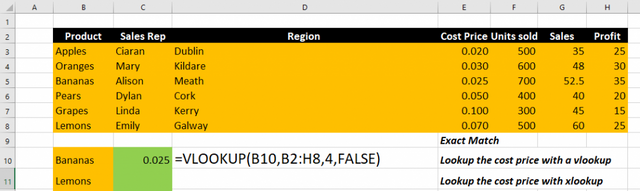Using the 5 steps above we can create our XLOOKUP.

Step 1: Select cell A11 and enter “=XLOOKUP( “

Step 2: Select the lookup value.  This is the value we wish to look up. In this case it is Lemons and we can find it in cell C11. Enter a comma.  “=XLOOKUP( A11,

Step 3: Select the lookup array.  This is the column or row that will contain the lookup value.  In this case the products are in cells B2:B8. Enter a comma.  “=XLOOKUP( A11, B2:B8, “

Step 4: Select the return array.  This is the column or row that will contain the values you wish to return.  In this case it’s the cost price column cells E2:E8.  “=XLOOKUP( A11, B2:B8, E2:E8

Step 5:  Close the formula with “)” and press enter =XLOOKUP( A11, B2:B8, E2:E8)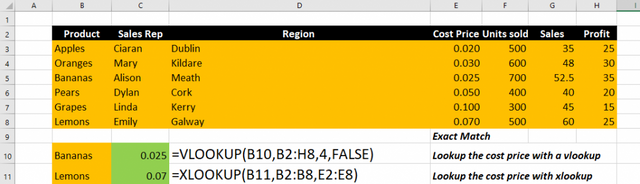## XLOOKUP removes limitations

With XLOOKUP as we enter a lookup array and a return array, excel is not trying to count columns in a table array as it does with VLOOKUP.  That means if we add new columns to our data, our XLOOKUP function will not break.  It also means you can place the return column to the left of the lookup column and easily carry out a look up to the left.  That’s two limitations of VLOOKUP removed.

In addition to this, with XLOOKUP you can carry out a horizontal lookup too.  With old Excel we needed a different function HLOOKUP to do this.

You can see in the image below, we have inserted a new column, D.  The old VLOOKUP no longer returns the correct column but all of our XLOOKUPs remain in tack.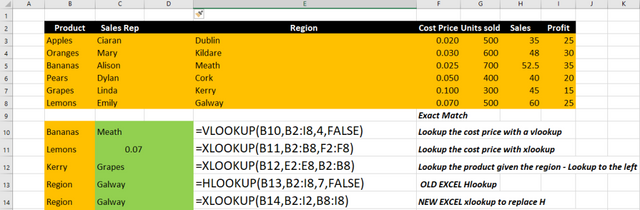We can also see in the image a look up to the left, where we have a Region and we need to lookup a product.  And we can also see XLOOKUP working like a HLOOKUP.

## Optional Parameters

The optional parameters include some new and exciting choices. These allow you set both the match type and the search mode.  The default value for Match mode is 0 – Exact match, therefore if you are using an exact match lookup you can omit this parameter.  The default value for Search mode is 1 – search first to last.  Once you want to search in this way, you can omit this parameter.

### Match mode

0 – Exact match

-1 – Exact match or next smaller

1 – Exact match or next larger

2 – Wildcard Character match

As the default match mode in XLOOKUP is Exact match. So when you are carrying out an exact match you can omit this value. This is the opposite in VLOOKUP where approximate match is the default. So if you want to carry out an exact match you need to ensure you select the range_lookup.

### Search mode

1 – search first to last

-1 - Search last to first

2 - Binary search (sorted ascending)

-2 Binary search (sorted descending)

The default search mode in XLOOKUP is from top to bottom, or first to last.  This is also the default and only search mode available in VLOOKUP.  Now with XLOOKUP we have new options.  We can search from the bottom to the top.  And we have Binary search options.  That’s even more limitations of VLOOKUP removed.

## Approximate Match Lookups with XLOOKUP

XLOOKUP allows for 4 different Match modes.  We have seen that exact match is the default and how it works, but we have yet to look at the other options.

Consider the following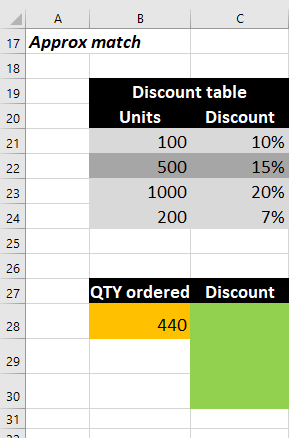We are given a discount table, showing the % discount given based on the units.  We wish to lookup the discount for the given QTY ordered.  In this example the QTY ordered is 440.

Looking at that table above we want to first lookup the discount for the given qty based on the next smallest item.  The formulas are shown in the image below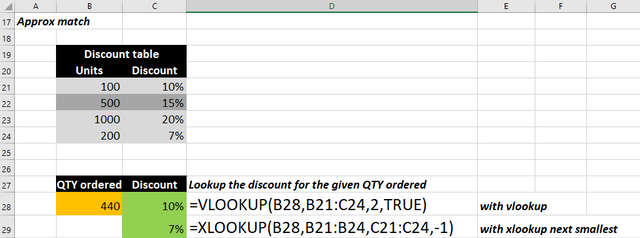Using the VLOOKUP the discount returned is 10%.  Note, this is not correct.  VLOOKUP requires sorting of the data from highest to lowest.  We have not sorted this table and so the wrong value is returned.  VLOOKUP stopped searching the column when it reached 500, as the lookup value is lower.

However, with XLOOKUP you do not need to sort the table and so the correct value of 7% is shown.  This is yet another limitation of VLOOKUP solved.

Note that for the match mode, we have selected -1 which is will return the next smallest values.  However, you can also carry out an XLOOKUP and return the next largest values.  To do this, select 1 as the match mode.

Study the image below as its shows the formula used to return an approximate match.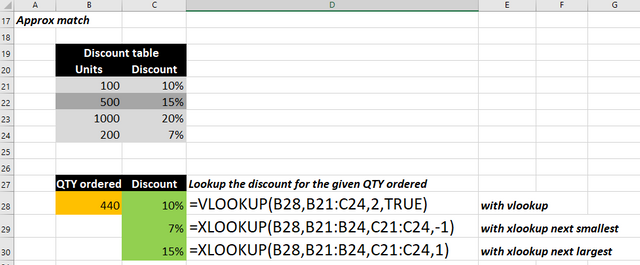## XLOOKUP Wildcard Searches

Wildcard searches are compatible with XLOOKUP.  ? will represent a 1 character wildcard while * represents multiple characters

Consider the following table of data.  We wish to lookup the Product “p?ars” and return the region.  This would be useful if you had a list of employees one of which is Linda which could also Lynda.  You could use a search “L?nda”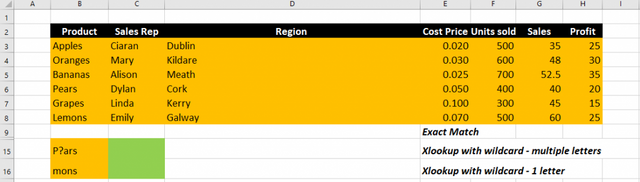We also wish to lookup the product that ends with the letters “mons”.  This product could have a number of letters before it and would therefore require the use of *

When carrying out a wildcard lookup using XLOOKUP you must specify in the match mode that it is wildcard lookup.  This is represented with 2 in the match mode menu.

Examine the image below, it shows the formulas used for the wildcard XLOOKUPs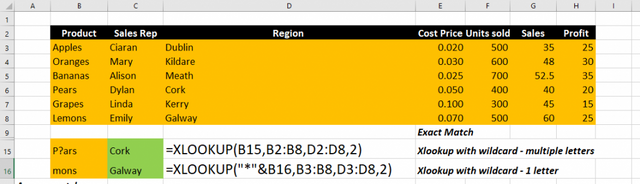You will note when is a wildcard search within text, the wildcard character “?” or “*” is places within the text.  However, if the wildcard search is at the beginning, or the end of the text, the you need to join it with &.

## Conclusion

XLOOKUP rocks.  It removes so many limitations of its predecessor VLOOKUP and it adds new functionality.  What we didn’t cover yet is the Search Modes but we have updated our Ultimate Excel 365 Formulas course with everything you need to know about using XLOOKUP.

# Learn and Earn

To earn rewards on this post, use the comments section below and answer the following questions

1. What do you think of XLOOKUP?  Do you think it is good?  Better than VLOOKUP?
2. How do you see your self apply XLOOKUP in your daily Excel use? Describe a use case in the comments below.

### Take a FREE course with usEnroll in FREE course NOW
##### Updated* Now include XLOOKUP
The Ultimate Excel Formulas Course Excel 365Explore The Ultimate Excel 365 Formulas course
Now there is value in Learning with The Excel Club and Learn and Earn activities.
By using Crypocurrency and blockchain technology , we are the first Excel, PowerBI and DAX blog in the world where you can earn while you learn.
CLICK here to find out how you can Earn while you Learn## Enjoyed this Excel Article? Before you leave, it’s only fair that you share

Cross posted from my blog with SteemPress : https://theexcelclub.com/xlookup-in-excel/
Sort Order:

1. I like this new xlookup. I think it is a great advancement. There are a lot of improvements over the existing vlookup. that makes it more functional than vlookup and its more versatile. I think it is way better than vlookup.
2. I have a number of tables where the requirement is to look to the left, or look down. I can now do both of these with the one function xlookup.

Thanks for the video. I had watched a few others, and you explained how to use xlookup very well. some people like to make it sound way more complex.

I am not on the right version of excel. Having not long learned how to use VLOOKUP, from your demonstration, this XLOOKUP function looks great. Better than Vlookup for sure. Thank you for sharing. how do I think I will use it, well I use vlookup to compile data into one table from other sources and I could use xlookup for this instead.

Congratulations @theexcelclub! You have completed the following achievement on the Steem blockchain and have been rewarded with new badge(s) :You distributed more than 14000 upvotes. Your next target is to reach 15000 upvotes.

You can view your badges on your Steem Board and compare to others on the Steem Ranking
If you no longer want to receive notifications, reply to this comment with the word `STOP`

Do not miss the last post from @steemitboard: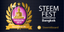The new SteemFest⁴ badge is ready